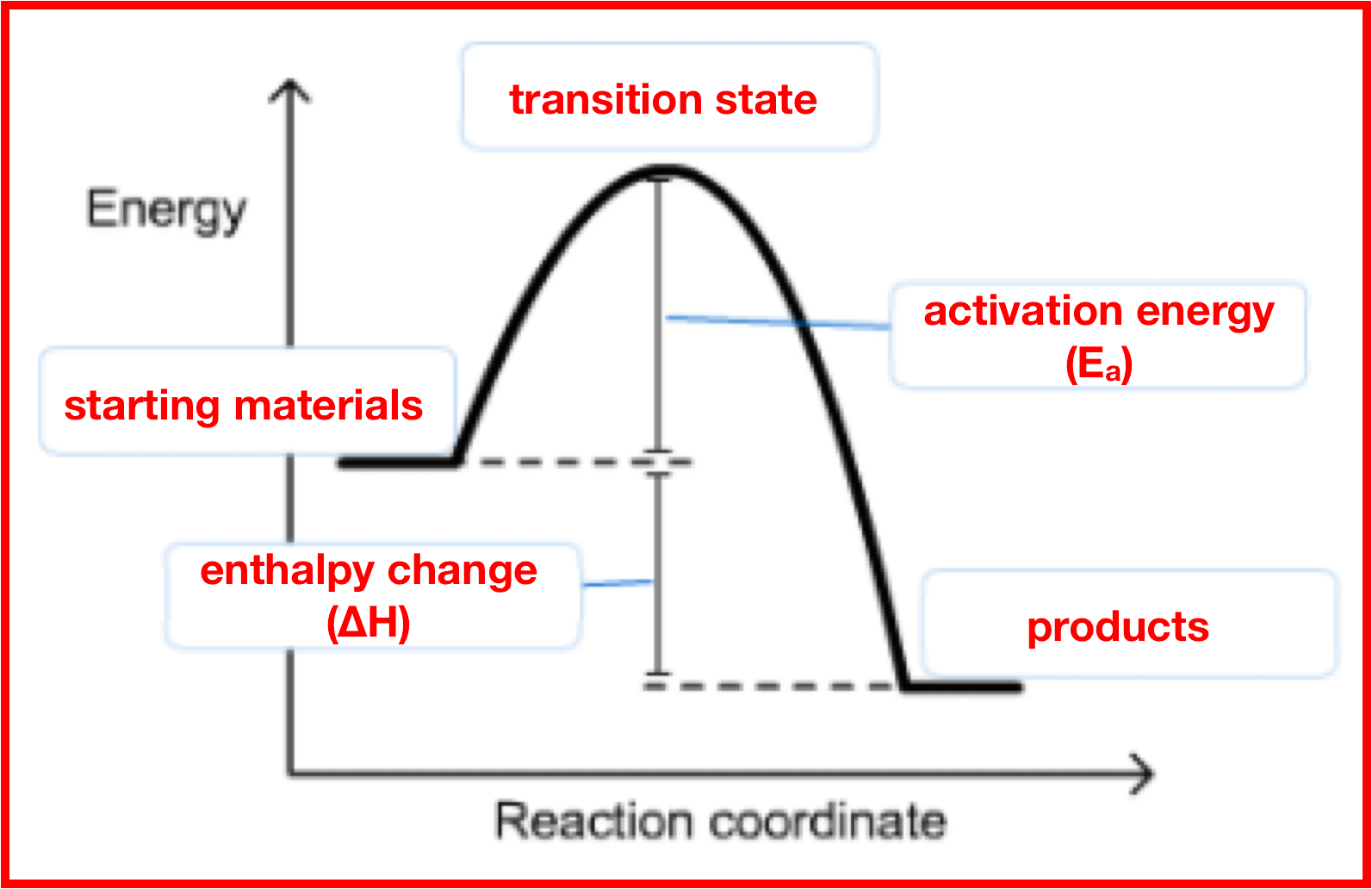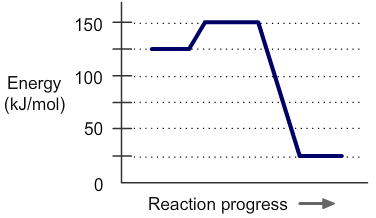# Problem: The diagram represents a spontaneous reaction. Use the diagram to answer the questions below. Is the reaction endothermic or exothermic? What is the activation energy of the reaction?

###### FREE Expert Solution

Recall that an energy diagram is usually read from left to rightThe components of a one-step energy diagram are:

88% (472 ratings)###### Problem Details

The diagram represents a spontaneous reaction. Use the diagram to answer the questions below.Is the reaction endothermic or exothermic?

What is the activation energy of the reaction?

Frequently Asked Questions

What scientific concept do you need to know in order to solve this problem?

Our tutors have indicated that to solve this problem you will need to apply the Energy Diagram concept. If you need more Energy Diagram practice, you can also practice Energy Diagram practice problems.

What professor is this problem relevant for?

Based on our data, we think this problem is relevant for Professor Kocerha's class at GS.